• Posts tagged "2048"

# Blog Archives

## R语言游戏之旅 游戏2048

R的极客理想系列文章，涵盖了R的思想，使用，工具，创新等的一系列要点，以我个人的学习和体验去诠释R的强大。

R语言作为统计学一门语言，一直在小众领域闪耀着光芒。直到大数据的爆发，R语言变成了一门炙手可热的数据分析的利器。随着越来越多的工程背景的人的加入，R语言的社区在迅速扩大成长。现在已不仅仅是统计领域，教育，银行，电商，互联网….都在使用R语言。

• 张丹(Conan), 程序员Java,R,PHP,Javascript
• weibo：@Conan_Z
• blog: http://blog.fens.me
• email: bsspirit@gmail.com

http://blog.fens.me/r-game-2048/150行代码写出游戏2048，哪种语言能实现？答案是R语言。虽然R语言并不适合做游戏开发，但是R语言中的向量计算，能极大地简化矩阵算法代码实现的复杂度，可以高效的完成计算任务。如果我们把游戏问题变成数学问题，那么R就是绝佳的工具。

1. 2048游戏介绍
2. 场景设计
3. 程序设计
4. R语言实现

## 1. 2048游戏介绍

2048是一款单人在线和移动端游戏，由19岁的意大利人Gabriele Cirulli于2014年3月开发。游戏任务是在一个网格上滑动小方块来进行组合，直到形成一个带有有数字2048的方块，它是滑块类游戏的一种电脑变体。作者开发这个游戏是为了测试自己是否有能力从零开始创造一款游戏，但游戏飙升的人气（不到1周内有400万访客）完全出乎他的预料。事实上，它已被称为网络上「最上瘾的东西」，华尔街日报将其评价为「属于数学极客的 Candy Crush」。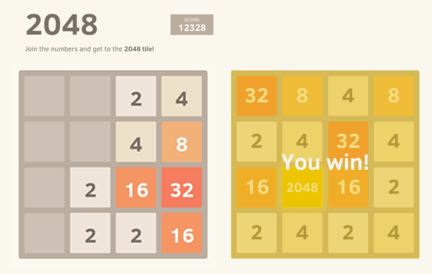## 2. 场景设计

2.1 需求分析

2048游戏，应该有3个场景：开机场景，游戏场景，结束场景。

• 开机场景：运行程序，在游戏前，给用户做准备，并提示如何操作游戏。
• 游戏场景：游戏运行中的场景。
• 结束场景：当用户胜利、失败或退出时的场景，并提示用户在游戏中的得分。

2.2 游戏规则

• 1. 开始游戏后，用户可以通过上(up)下(down)左(left)右(right)键，来控制数字的移动。
• 2. 如果两个相同的数字在移动中碰撞，则它们会合并为一个方块，且所带数字变为两者之和。
• 3. 每次移动时，会有一个值为2或者4的新数字出现。
• 4. 当用户按键操作，数字的顺序未发生变化时，则不会生成新数字，视为无效的按键操作。
• 5. 当画布格子被数字填满时，而在上下左边方向，无可合并的数字时，则游戏失败。

2.3 业务流程

• 打开程序时，用户首先看到开机场景，按任意键后进入游戏场景。
• 在游戏场景，当游戏失败，进入结束场景；按q键，则直接游戏失败。
• 在结束场景，按空格回到开机场景；按q键，则直接能出软件。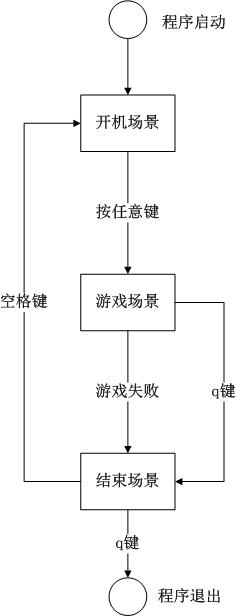2.4 游戏原型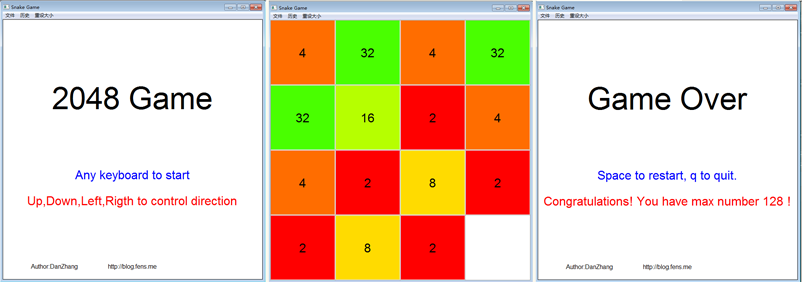## 3. 程序设计

3.1 游戏场景

• 开机场景，是静态的，我们可以提前生成好这块画布存储起来，也可以当用户切换时再临时生成，性能开销不大。
• 游戏场景，是动态的，每进行一次用户的交互行为或按时间刷新时，都需要求重新绘制画布，让游戏场景通过绑定事件来生成画布。
• 结束场景，是动态的，在结束场景会显示当次游戏的得分，需要在切换时临时生成。

3.2 游戏对象

• 画布对象：用矩阵来描述。
• 画布中的数字：用矩阵中的数字值来表示。
• 画布的背景色：用矩阵中的数字值来表示。

``````
[,1] [,2] [,3] [,4]
[1,]    4   32    4   32
[2,]   32   16    2    4
[3,]    4    2    8    2
[4,]    2    8    2    0
``````

3.3 游戏事件

• 键盘事件：全局事件，用户通过键盘输入，而触发的事件，比如，上下左右控制蛇的移动方向。
• 碰撞事件：如果两个相同的数字在移动中碰撞，则它们会合并为一个数字。

3.4 游戏控制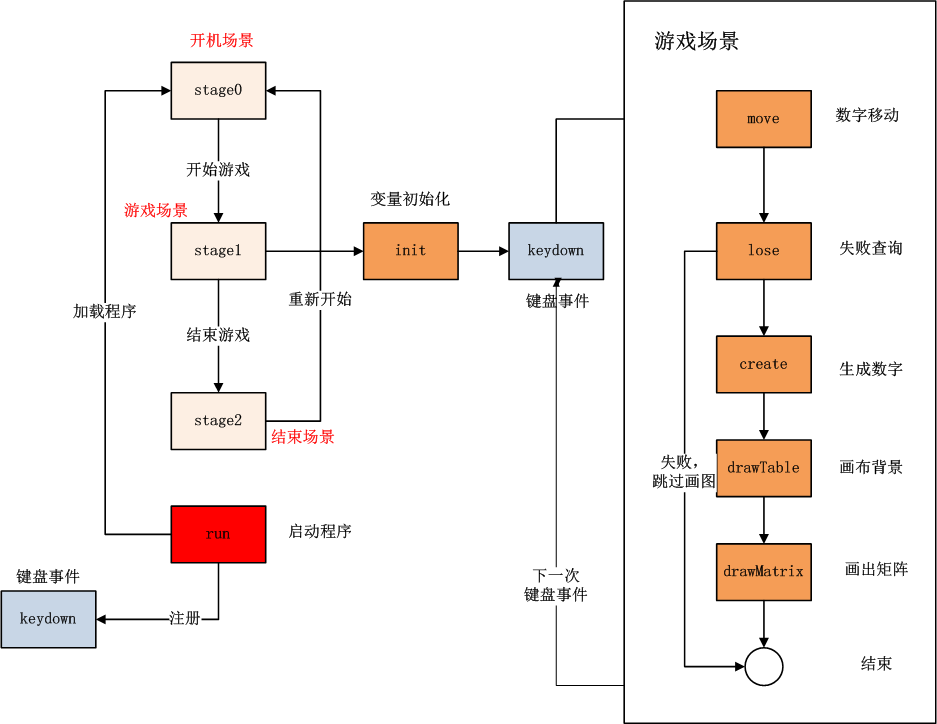• run():启动程序。
• keydown():监听键盘事件，锁定线程。
• stage0():创建开机场景，可视化输出。
• stage1():创建游戏场景，可视化输出。
• stage2():创建结束场景，可视化输出。
• init():打开游戏场景时，初始化游戏变量。
• create():判断并生成数字。
• move():移动数字。
• lose():失败查询，判断当画布格子是否被被数字填满时，且不能合并的数字时，进行结束场景。
• drawTable():绘制游戏背景。
• drawMatrix():绘制游戏矩阵。

## 4. R语言实现

4.1 数字移动函数 move()

2048游戏算法上最复杂的操作，就是数字移动。在4*4的矩阵中，数字会按上下左右四个方向移动，相同的数字在移动中碰撞时会进行合并。这个算法是2048游戏的核心算法，我们的程序要保证数字合并正确性。

``````
> moveFun<-function(x,dir){
+   if(dir == 'right') x<-rev(x)
+
+   len0<-length(which(x==0)) # 0长度
+   x1<-x[which(x>0)] #去掉0
+   pos1<-which(diff(x1)==0) # 找到挨着相等的元素的位置
+
+   if(length(pos1)==3){ #3个索引
+     pos1<-pos1[c(1,3)]
+   }else if(length(pos1)==2 && diff(pos1)==1){ #2个索引
+     pos1<-pos1
+   }
+
+   x1[pos1]<-x1[pos1]*2
+   x1[pos1+1]<-0
+
+   x1<-x1[which(x1>0)] #去掉0
+   x1<-c(x1,rep(0,4))[1:4] #补0，取4个
+
+   if(dir == 'right') x1<-rev(x1)
+   return(x1)
+ }
``````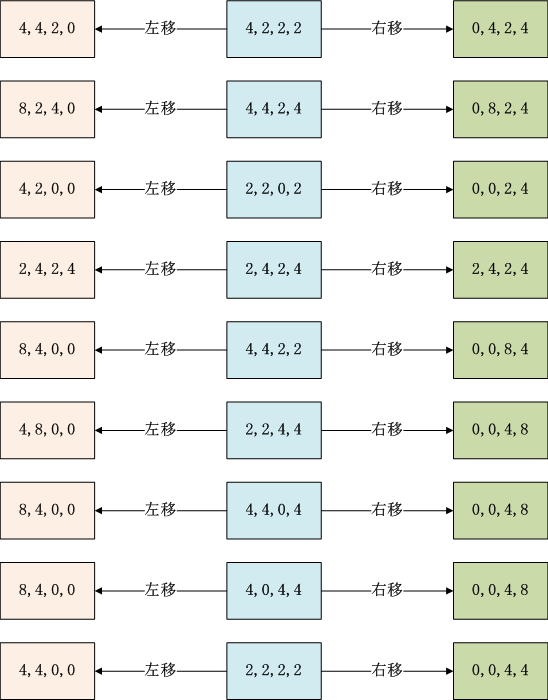``````
> library(testthat)
> x<-c(4,2,2,2)
> expect_that(moveFun(x,'left'), equals(c(4,4,2,0)))
> expect_that(moveFun(x,'right'), equals(c(0,4,2,4)))

> x<-c(4,4,2,4)
> expect_that(moveFun(x,'left'), equals(c(8,2,4,0)))
> expect_that(moveFun(x,'right'), equals(c(0,8,2,4)))

> x<-c(2,2,0,2)
> expect_that(moveFun(x,'left'), equals(c(4,2,0,0)))
> expect_that(moveFun(x,'right'), equals(c(0,0,2,4)))

> x<-c(2,4,2,4)
> expect_that(moveFun(x,'left'), equals(c(2,4,2,4)))
> expect_that(moveFun(x,'right'), equals(c(2,4,2,4)))

> x<-c(4,4,2,2)
> expect_that(moveFun(x,'left'), equals(c(8,4,0,0)))
> expect_that(moveFun(x,'right'), equals(c(0,0,8,4)))

> x<-c(2,2,4,4)
> expect_that(moveFun(x,'left'), equals(c(4,8,0,0)))
> expect_that(moveFun(x,'right'), equals(c(0,0,4,8)))

> x<-c(4,4,0,4)
> expect_that(moveFun(x,'left'), equals(c(8,4,0,0)))
> expect_that(moveFun(x,'right'), equals(c(0,0,4,8)))

> x<-c(4,0,4,4)
> expect_that(moveFun(x,'left'), equals(c(8,4,0,0)))
> expect_that(moveFun(x,'right'), equals(c(0,0,4,8)))

> x<-c(4,0,4,2)
> expect_that(moveFun(x,'left'), equals(c(8,2,0,0)))
> expect_that(moveFun(x,'right'), equals(c(0,0,8,2)))

> x<-c(2,2,2,2)
> expect_that(moveFun(x,'left'), equals(c(4,4,0,0)))
> expect_that(moveFun(x,'right'), equals(c(0,0,4,4)))

> x<-c(2,2,2,0)
> expect_that(moveFun(x,'left'), equals(c(4,2,0,0)))
> expect_that(moveFun(x,'right'), equals(c(0,0,2,4)))
``````

4.2 其他函数实现

``````
# 开机画图
stage0=function(){
callSuper()
plot(0,0,xlim=c(0,1),ylim=c(0,1),type='n',xaxs="i", yaxs="i")
text(0.5,0.7,label=name,cex=5)
text(0.5,0.4,label="Any keyboard to start",cex=2,col=4)
text(0.5,0.3,label="Up,Down,Left,Rigth to control direction",cex=2,col=2)
text(0.2,0.05,label="Author:DanZhang",cex=1)
text(0.5,0.05,label="http://blog.fens.me",cex=1)
}
``````

``````
# 结束画图
stage2=function(){
callSuper()
info<-paste("Congratulations! You have max number",max(m),"!")
print(info)

plot(0,0,xlim=c(0,1),ylim=c(0,1),type='n',xaxs="i", yaxs="i")
text(0.5,0.7,label="Game Over",cex=5)
text(0.5,0.4,label="Space to restart, q to quit.",cex=2,col=4)
text(0.5,0.3,label=info,cex=2,col=2)
text(0.2,0.05,label="Author:DanZhang",cex=1)
text(0.5,0.05,label="http://blog.fens.me",cex=1)
}
``````

``````
# 键盘事件，控制场景切换
keydown=function(K){
callSuper(K)

if(stage==1){ #游戏中
if(K == "q") stage2()
else {
if(tolower(K) %in% c("up","down","left","right")){
e\$dir<<-tolower(K)
print(e\$dir)
stage1()
}
}
return(NULL)
}
return(NULL)
}
``````

``````
# 初始化变量
init = function(){
callSuper()  # 调父类

e\$max<<-4 # 最大数字
e\$step<<-1/width #步长
e\$dir<<-'up'
e\$colors<<-rainbow(14) #颜色
e\$stop<<-FALSE #不满足移动条件

create()
}
``````

``````
# 随机产生一个新数字
create=function(){
if(length(index(0))>0 & !e\$stop){
e\$stop<<-TRUE
one<-sample(c(2,4),1)
idx<-ifelse(length(index(0))==1,index(0),sample(index(0),1))
m[idx]<<-one
}
}
``````

``````
#失败条件
lose=function(){

# 判断是否有相邻的有重复值
near<-function(x){
length(which(diff(x)==0))
}

# 无空格子
if(length(index(0))==0){
h<-apply(m,1,near)  # 水平方向
v<-apply(m,2,near) # 垂直方向

if(length(which(h>0))==0 & length(which(v>0))==0){
fail("No free grid.")
return(NULL)
}
}
}
``````

``````
# 画布背景
drawTable=function(){
if(isFail) return(NULL)
plot(0,0,xlim=c(0,1),ylim=c(0,1),type='n',xaxs="i", yaxs="i")
abline(h=seq(0,1,e\$step),col="gray60") # 水平线
abline(v=seq(0,1,e\$step),col="gray60") # 垂直线
}
``````

``````
# 根据矩阵画数据
drawMatrix=function(){
if(isFail) return(NULL)
a<-c(t(m))
lab<-c(a[13:16],a[9:12],a[5:8],a[1:4])

d<-data.frame(x=rep(seq(0,0.95,e\$step),width),y=rep(seq(0,0.95,e\$step),each=height),lab=lab)
df<-d[which(d\$lab>0),]
points(df\$x+e\$step/2,df\$y+e\$step/2,col=e\$colors[log(df\$lab,2)],pch=15,cex=23)
text(df\$x+e\$step/2,df\$y+e\$step/2,label=df\$lab,cex=2)
}
``````

``````
# 游戏场景
stage1=function(){
callSuper()

move()
lose()
create()

drawTable()
drawMatrix()
}
``````

``````
source(file="game.r") #加载游戏框架

# Snake类，继承Game类
G2048<-setRefClass("G2048",contains="Game",

methods=list(

# 构造函数
initialize = function(name,debug) {
callSuper(name,debug) # 调父类

name<<-"2048 Game"
width<<-height<<-4
},

# 初始化变量
init = function(){
callSuper()  # 调父类

e\$max<<-4 # 最大数字
e\$step<<-1/width #步长
e\$dir<<-'up'
e\$colors<<-rainbow(14) #颜色
e\$stop<<-FALSE #不满足移动条件

create()
},

# 随机产生一个新数字
create=function(){
if(length(index(0))>0 & !e\$stop){
e\$stop<<-TRUE
one<-sample(c(2,4),1)
idx<-ifelse(length(index(0))==1,index(0),sample(index(0),1))
m[idx]<<-one
}
},

#失败条件
lose=function(){

# 判断是否有相邻的有重复值
near<-function(x){
length(which(diff(x)==0))
}

# 无空格子
if(length(index(0))==0){
h<-apply(m,1,near)  # 水平方向
v<-apply(m,2,near) # 垂直方向

if(length(which(h>0))==0 & length(which(v>0))==0){
fail("No free grid.")
return(NULL)
}
}
},

# 方向移动
move=function(){

# 方向移动函数
moveFun=function(x){
if(e\$dir %in% c('right','down')) x<-rev(x)

len0<-length(which(x==0)) # 0长度
x1<-x[which(x>0)] #去掉0
pos1<-which(diff(x1)==0) # 找到挨着相等的元素的位置

if(length(pos1)==3){ #3个索引
pos1<-pos1[c(1,3)]
}else if(length(pos1)==2 && diff(pos1)==1){ #2个索引
pos1<-pos1
}

x1[pos1]<-x1[pos1]*2
x1[pos1+1]<-0

x1<-x1[which(x1>0)] #去掉0
x1<-c(x1,rep(0,4))[1:4] #补0，取4个

if(e\$dir %in% c('right','down')) x1<-rev(x1)
return(x1)
}

last_m<-m
if(e\$dir=='left')  m<<-t(apply(m,1,moveFun))
if(e\$dir=='right') m<<-t(apply(m,1,moveFun))
if(e\$dir=='up')    m<<-apply(m,2,moveFun)
if(e\$dir=='down')  m<<-apply(m,2,moveFun)

e\$stop<<-ifelse(length(which(m != last_m))==0,TRUE,FALSE)
},

# 画布背景
drawTable=function(){
if(isFail) return(NULL)
plot(0,0,xlim=c(0,1),ylim=c(0,1),type='n',xaxs="i", yaxs="i")
abline(h=seq(0,1,e\$step),col="gray60") # 水平线
abline(v=seq(0,1,e\$step),col="gray60") # 垂直线
},

# 根据矩阵画数据
drawMatrix=function(){
if(isFail) return(NULL)
a<-c(t(m))
lab<-c(a[13:16],a[9:12],a[5:8],a[1:4])

d<-data.frame(x=rep(seq(0,0.95,e\$step),width),y=rep(seq(0,0.95,e\$step),each=height),lab=lab)
df<-d[which(d\$lab>0),]
points(df\$x+e\$step/2,df\$y+e\$step/2,col=e\$colors[log(df\$lab,2)],pch=15,cex=23)
text(df\$x+e\$step/2,df\$y+e\$step/2,label=df\$lab,cex=2)
},

# 游戏场景
stage1=function(){
callSuper()

move()
lose()
create()

drawTable()
drawMatrix()
},

# 开机画图
stage0=function(){
callSuper()
plot(0,0,xlim=c(0,1),ylim=c(0,1),type='n',xaxs="i", yaxs="i")
text(0.5,0.7,label=name,cex=5)
text(0.5,0.4,label="Any keyboard to start",cex=2,col=4)
text(0.5,0.3,label="Up,Down,Left,Rigth to control direction",cex=2,col=2)
text(0.2,0.05,label="Author:DanZhang",cex=1)
text(0.5,0.05,label="http://blog.fens.me",cex=1)
},

# 结束画图
stage2=function(){
callSuper()
info<-paste("Congratulations! You have max number",max(m),"!")
print(info)

plot(0,0,xlim=c(0,1),ylim=c(0,1),type='n',xaxs="i", yaxs="i")
text(0.5,0.7,label="Game Over",cex=5)
text(0.5,0.4,label="Space to restart, q to quit.",cex=2,col=4)
text(0.5,0.3,label=info,cex=2,col=2)
text(0.2,0.05,label="Author:DanZhang",cex=1)
text(0.5,0.05,label="http://blog.fens.me",cex=1)
},

# 键盘事件，控制场景切换
keydown=function(K){
callSuper(K)

if(stage==1){ #游戏中
if(K == "q") stage2()
else {
if(tolower(K) %in% c("up","down","left","right")){
e\$dir<<-tolower(K)
stage1()
}
}
return(NULL)
}
return(NULL)
}

)
)

# 封装启动函数
g2048<-function(){
game<-G2048\$new()
game\$initFields(debug=TRUE)
game\$run()
}

# 启动游戏
g2048()
``````

http://blog.fens.me/r-game-2048/